American Journal of Modern Physics
Volume 5, Issue 4, July 2016, Pages: 58-64

Time, Space, Gravity and Spacetime Based on Yangton & Yington Theory, and Spacetime Shrinkage Versus Universe Expansion

Edward T. H. Wu

Solar Buster Corporation, Los Angeles, USA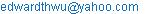Edward T. H. Wu. Time, Space, Gravity and Spacetime Based on Yangton & Yington Theory, and Spacetime Shrinkage Versus Universe Expansion. American Journal of Modern Physics. Vol. 5, No. 4, 2016, pp. 58-64. doi: 10.11648/j.ajmp.20160504.13

Received: May 12, 2016; Accepted: May 30, 2016; Published: July 13, 2016

Abstract: Wu’s Particle is proposed as the building block of all Matters, therefore Time and Length can be measured by the Period and Size of the circulation of Wu’s Particle. For a local event, object and process, the Time, Length and Velocity measured by the Period and Size of Wu’s Particle have equal values as that of the identical events, objects and processes occurred at different locations measured by the corresponding Periods and Sizes of Wu’s Particles. However, for a remote event, object and process, the Time, Length and Velocity change with the Period and Size of Wu’s Particle at observation. For an event, object and process occurred in a large Gravitational Field and an ancient Universe, Time runs slower, Length gets longer and Velocity is smaller observed on Earth. As a result, light travels at lower speed with lower frequency and larger wavelength from a large Gravitational Field known as Gravitational Red Shift; also from a star of a few millions light years away known as Cosmological Red Shift. Spacetime is a four dimensional system based on the Period and Size of Wu’s Particle. Since the Period and Size of Wu’s Particle change with Gravitational Field and Concentration of Higgs Bosons, Spacetime is a function of Gravitational Field and Concentration of Higgs Bosons. In Wu’s Particle, Time is proportional to 3/2 power of Length which is named as “Wu’s Spacetime Theory”. Furthermore, instead of “Universe Accelerating Expansion Theory” which is based on the non-existing Dark Energy, “Spacetime Accelerating Shrinkage Theory” is proposed to explain the accelerating expansion of the Universe.

Keywords: Yangton, Yington, Photon, Wu’s Particle, Higgs Boson, Particle Radiation, Gravitational Force, Gravitational Field, Time, Space, Spacetime, Black Hole, Gravitational Red Shift, Cosmological Red Shift, Universe Expansion, Dark Energy

Contents

1. Introduction

The meaning of "Time" and "Length" are confusing to most of the people even scientists. "Time" is a measurement of the duration of an event by the cycles of a "Unit Time", the period of a repeating physical process; and "Length" on the other hand is a measurement of the size of an object by the multiplication of a "Unit Length", a specific amount of distance.

Recently, a hypothetical theory of a Yangton and Yington circulating pair  has been proposed by Edward T. H. Wu successfully in explanation of a variety of physical phenomena such as:

Creation of Universe based on Yangton and Yington Theory 

Photon Structure and Particle & Wave Properties 

Wu’s Particle as the building block of all Matters 

Einstein Theory E = MC2 and Mechanism interpreted by Yangton and Yington Theory 

Black Hole as the grave yard of Yangton and Yington particles 

Electron and Positron Structures and Electromagnetic Force 

Higgs Boson, String Structure and Gravitational Force 

Subatomic Particle Structures and Four Basic Forces 

Force of Creation and Unified Field Theory 

Mass and Energy of Photon and Wu’s Particle 

Constant Light Speed and Mechanism 

Black Body Radiation and Wu’s Particle 

Vision of Light and Light Speed 

Light Speed and Anti Special Relativity 

Particle Radiation and Contact Interaction Theory 

Gravitational Waves and Higgs Boson Radiation 

In this paper, the definitions and relationships between Space, Time, Gravity and Spacetime  will be discussed. "Time" will be measured by the period of the circulation of Wu’s Particle and "Length" will be measured by the size of the circulation of Wu’s Particle. Correlation between "Time" and "Length" will also be studied. Furthermore, instead of "Universe Accelerating Expansion Theory" based on the non-existing "Dark Energy", "Spacetime Accelerating Shrinkage Theory" based on Wu’s Particle, will be proposed to explain the accelerating expansion of the Universe.

2. Yangton & Yington Pair

It is proposed by Edward T. H. Wu  that a pair of super fine particles, Yangton and Yington with an inter-attractive "Force of Creation" (Fig. 1), can be spontaneously formed in the Universe at anytime and anywhere. However, due to the enforcement of the inter-attractive "Force of Creation", Yangton and Yington immediately recombine and destroy each other such that something becomes nothing and everything disappeared again in the empty space.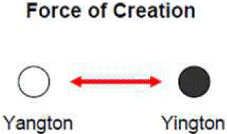Fig. 1. A Yangton and YIngton Pair and Force of Creation.

Not until the Big Bang explosion , Yangton and Yington pair became permanent existence by absorbing "Energy of Formation" and started moving simultaneously after each other in a circular orbit balanced by the centrifugal force against the inter-attractive "Force of Creation". This Yangton and Yington circulating pair is known as "Wu’s Particle" (Fig. 2).

2.1. Photon

Photon is a free "Wu’s Particle", a Yangton and Yington circulating pair that travels in different Matters at different speeds.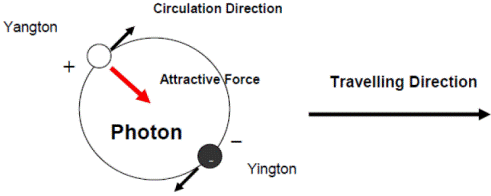Fig. 2. Wu’s Particle and Photon formed by Yangton and Yington Circulating Pair.

Because of the inter-attractive force between Yangton and Yington, it is assumed that Yangton carries one positive unit-charge and Yington carries one negative unit-charge and together they form an electric dipole. These unit-charges are the basic units of the normal electric charges that are carried by Electrons and Protons except in a much smaller scale.

Resulting from the circulation of the Yangton and Yington pair, or in another word the rotation of the electric dipole inside the Photon, Electromagnetic Wave can be generated and carried by a free Photon to far distance at light speed (Fig. 3). From a macroscopic point of view, Photon can be considered as a neutral particle, simply because any external electric force applied on Yangton can be cancelled completely by the counter electric force applied on Yington due to their ultra fine particle sizes and circulation orbit.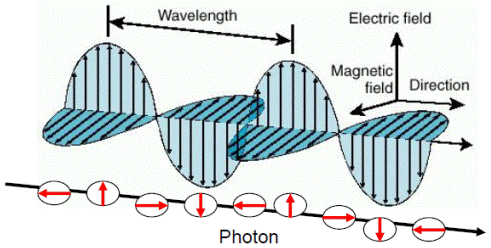Fig. 3. Electromagnetic wave of a Photon generated along traveling.

2.2. Wu’s Particle

"Wu’s Particle", a still Yangton and Yington circulating pair forms the basic building block of all Matters  . With the preference of orientation due to the attractive force between Yangton and Yington particles and the dipoles in the adjacent "Wu’s Particles", it is proposed that a variety of subatomic particles can be formed by "Wu’s Particle" such as Higgs Boson and Neutron with String and Ring Structures respectively (Fig. 4).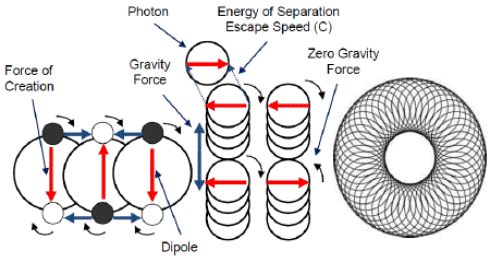Fig. 4. Wu’s Particles stack up in a preferred direction to form String and Ring Structures.

3. Gravity

3.1. Gravitational Force and Higgs Boson

A Higgs Boson with String Structure made of "Wu’s Particle" has strong dipoles at ends and sides (Fig. 4). When two Higgs Bosons come together with the same circulation direction, attractive force can be generated at the contact points either at the ends or on the sides of the String Structures . But there is no interference for those with the opposite circulation directions (Fig. 5). This attractive force known as the Gravitational Force  can be commonly found in the Universe between substances.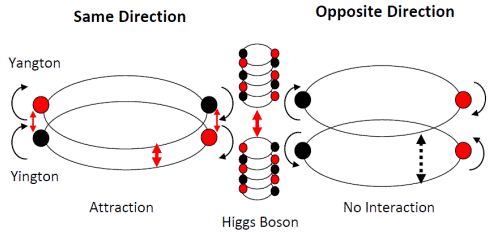Fig. 5. Gravitational Force between two Higgs Bosons.

3.2. Higgs Boson Radiation and Interaction Theory

Newton’s Law of Universal Gravitation  gives the total amount of Gravitational Force generated between two objects. However, in the past three centuries there is no explanation about how the Gravitational Force propagates through the space and how it reacts with the target object. Not until recently Edward T. H. Wu proposed a "Particle Radiation and Interaction Theory"  and has successfully explained the mechanisms and the processes of the phenomena.

Like Photons emitted from a substance by absorbing thermal energy to overcome its "Energy of Separation" , it is believed that Higgs Bosons with String Structures can also be emitted from a substance by absorbing thermal energy to overcome its Gravitational Force . In the "Higgs Boson Radiation" process, the concentration of emitted Higgs Bosons should be proportional to the total amount of Higgs Bosons in the parent object, the mass of the parent object (m1). Along with the propagation process, the concentration of emitted Higgs Bosons will be diluted by a factor that is inversely proportional to the square of the distance (1/r2) from the parent object (Fig. 6) which is known as "Inverse Square Law" .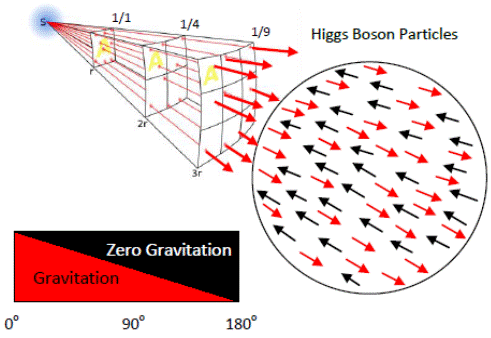Fig. 6. Inverse Square Law and Contact Interaction between Higgs Bosons of same circulation direction.

It is obvious that only those Higgs Bosons in line of sight with target object can completely reach the target object to make "Contact Interaction" without blocking by other objects. Also, because the Higgs Bosons in the target object can have circulation directions at angels anywhere from 0° to 180° away from that of incoming Higgs Bosons, therefore only those Higgs Bosons in the target object having circulation directions that are in parallel or partially parallel to the incoming Higgs Bosons can make "Contact Interaction" and contribute to the attractive force at the contact points, that is 50% of the total amount of Higgs Bosons (Fig. 6) in the target object (m2).

Since the total Gravitational Force generated at the contact points at any time should be proportional to the concentration of the Higgs Bosons at the contact points emitted from the parent object (m1/r2), and also the total amount of the Higgs Bosons in the target object (m2), therefore a formula such as Newton’s Law of Universal Gravitation can be derived and Gravitational Force (Fg) can be calculated as follows:

Fg = G (m1m2/r2)    (1)

Where G is the gravitational constant (6.674×10−11 N m2 kg-2).

Thus the Gravitational Field (g1) applied on a target object of Unit Mass from any parent object m1 can be expressed as follows:

g1 = G (m1/r2)

Therefore, Gravitational Field (g) at any point in the Universe is proportional to the concentration of Higgs Bosons particles (∑m/r2) emitted from all parent objects to that position.

g = G (∑m/r2)    (2)

4. Circulating Pair

Fig. 7 is a schematic picture of Wu’s Particle, a Yangton and Yington Circulating Pair. The central acceleration (ac) can be calculated as follows:

ac = dV/dt = (V1 dS/r)/dt = V1(dS/dt)/r = V2/r

Because,

dP = Fcdt = kdV

Fc = k(dV/dt) = kac

Where Fc is the central force and k is a constant.

Therefore,

Fc ∞ ac ∞ V2/r

Because,

F attraction ∞ 1/(2r)2

Also,

Fc = F attraction

Therefore,

V2/r = constant/4r2

And,

V2r = constant   (3)

Where V is the velocity of circulation and r is the radius of the circulation orbit.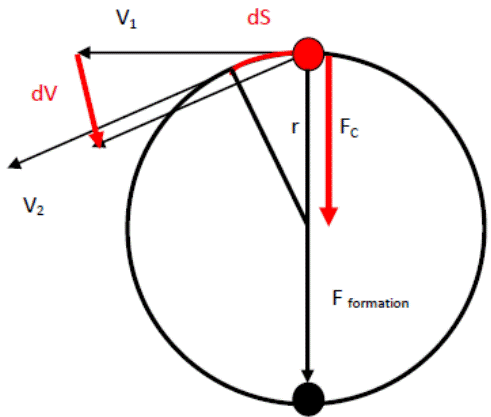Fig. 7. Theory of Circulating Pair.

5. Time

5.1. Wu’s Time

Since Wu’s Particle is proposed as the building block of all Matters, the duration of an event "Wu’s Time" (tw) can be measured by the "Period" of the circulation of Wu’s Particle "Wu’s Unit Time" (tyy) at observation.

Wu’s Unit Time (tyy) increases with Gravitational Field and decreases with Age of Universe. When Gravitational Field increases, the attractive force applied on the Higgs Boson also increases, thus the circulation orbit (2r) of Wu’s Particle is getting bigger. According to equation (3), V2r is always a constant for an attractive circulating pair such as Wu’ Particle, therefore circulating speed (V) gets smaller and the circulating period tyy (tyy = 2πr/V) of Wu’s Particle becomes longer. On the other hand, when Universe grows older, circulation orbit gets smaller and circulating speed of Wu’s Particle becomes larger, therefore the circulating period tyy of Wu’s Particle gets shorter.

It is obvious that Wu’s Time (tw) of a local event measured by Wu’s Unit Time (tyy), the "Period" of Wu’s Particle at the same position should have the equal value as that of the identical events occurred at different locations measured by the corresponding "Periods" of Wu’s Particles no matter of Gravitational Field and Age of Universe,

tw = a tyy   (4)

Where "a" is a constant.

5.2. Normal Time

Normal Time (tn) is the duration of an event measured by the Normal Unit Time (ts) which is defined by the period of a specific physical process. According to equation (4), for any event, tn and ts each contains constant amount of Wu’s Unit Time (tyy) no matter of Gravitaional Field and Age of Universe, therefore,

tn = btyy

ts = ctyy

tn = (b/c)ts

Giving,

d = b/c

Therefore,

tn = dts   (5)

Where "b", "c" and "d" are constants.

Therefore for any event, the Normal Time (tn) of the event measured by the Normal Unit Time (ts) at the same position will always have a constant value no matter of the Gravitational Field and Age of Universe. For example, Caesium oscillator has oscillation period 1/9,192,631,770 seconds on Earth . The same oscillator moves to Mars will still have the same oscillation period of 1/9,192,631,770 seconds, however the "Second" on Mars is "Mars Second" instead of "Earth Second".

6. Space

6.1. Wu’s Length

Similar to "Wu’s Time", the length of an object "Wu’s Length" can also be measured by the "Size" of the circulation of Wu’s Particle "Wu’s Unit Length" (lyy) at observation.

Wu’s Unit Length (lyy) increases with Gravitational Field and decreases with Age of Universe. When Gravitational Field increases, the attractive force applied on the Higgs Boson also increases, thus the circulation orbit (2r = lyy) of Wu’s Particle is getting bigger, therefore the size of Wu’s Particle lyy becomes bigger. On the other hand, when Universe grows older, the circulation speed is getting faster. According to equation (3), V2r is always a constant, therefore the circulation orbit of Wu’s Particle is smaller, and the size of Wu’s Particle lyy also becomes smaller.

It is obvious that Wu’s Length (lw) of an object measured by Wu’s Unit Length (lyy), the "Size" of Wu’s Particle at the same position should have the equal value as that of the identical objects occurred at different locations measured by the corresponding "Sizes" of Wu’s Particles no matter of Gravitational Field and Age of Universe,

lw = elyy   (6)

Where "e" is a constant.

6.2. Normal Length

Normal Length (ln) is the length of an object measured by the Normal Unit Length (ls) which is defined by the size of a specific physical object. According to equation (6), for any object, ln and ls each contains constant amount of Wu’s Unit Length (tyy) no matter of Gravitaional Field and Age of Universe, therefore,

ln = mlyy

ls = nlyy

ln = (m/n)ls

Giving,

p = m/n

Therefore,

ln = pls   (7)

Where "m", "n" and "p" are constants.

Therefore for any object, the Normal Length (ln) measured by the Normal Unit Length (ls) at the same position will always have a constant value no matter of the Gravitational Field and Age of Universe. For example, a "one foot" ruler has length on Earth 30.48 cm. The same ruler moves to Mars will still have the same length 30.48 cm, however the "cm" on Mars is "Mars cm" instead of "Earth cm".

7. Spacetime

Spacetime [tyy, lyy]  is a four dimensional system [x, y, z, t] based on the Wu’s Unit Time (tyy), the period of Wu’ Particle, and the Wu’s Unit Length (lyy), the size of Wu’s Particle, at a reference point in the Universe.

According to Wu’s Yangton and Yington Theory, both the Wu’s Unit Time (tyy) and Wu’s Unit Length (lyy) are functions of Gravitational Field (g). Also Gravitational Field (g) is a function of the concentration of Higgs Bosons (CHiggs), therefore Spacetime [tyy, lyy] is a four dimensional system of Gravitational Field [tyy(g), lyy(g)] and also a four dimensional system of the concentration of Higgs Bosons [tyy(CHiggs), lyy(CHiggs)] at a reference point in the Universe.

7.1. Wu’s Spacetime Theory

The circulation period (tyy) and the size (lyy) of Wu’s Particle are related to each other as follows:

Because of (3),

V2r = constant

And,

T = 2πr/V

T2 = 4π2r2/V2 = 4π2r3/V2r = 4k1 π2r3

T = k2r3/2

Where T is the period tyy, k1 and k2 are constants.

Therefore,

tyy = k3lyy3/2   (8)

Where lyy = 2r and k3 is a constant.

Wu’s Unit Time (tyy) is proportional to 3/2 power of Wu’s Unit Length (lyy). This is named as "Wu’s Spacetime Theory".

7.2. Relative Velocity

For an identical moving process at any Gravitational Fields and Ages of Universe, its relative speed is constant.

Because of (4), (5), (6) & (7)

tn = x ts

ln = y ls

ts = m tyy

ls = n lyy

V = dln/dtn = (dy/dx) ls/ts = (dy/dx) (n/m) lyy/tyy

Because dy/dx, n and m are constants,

Vr = V/(lyy/tyy) = (dy/dx) (n/m) = Constant(9)

Where Vr is relative velocity and V is absolute velocity.

In another word, for an identical moving process in any Spacetime, Relative Velocity is always a constant measured by the observer in stationary to the Spacetime.

7.3. Absolute Velocity

Because of (8),

lyy/tyy = k4lyy-1/2(10)

Where k4 is a constant.

Also (9) & (10),

V ∞ lyy-1/2     (11)

For a moving process in a high Gravitational Field or an ancient Universe, the size (lyy) of Wu’s Particle is bigger, but the Absolute Velocity (V) of the process is smaller.

7.4. Photon and Spacetime

As to a Photon,

ν = 1/tyy

Because of (8),

ν ∞ lyy-3/2   (12)

Because of (11),

C ∞ lyy-1/2

Because,

λ = C/ν

Therefore,

λ ∞ lyy   (13)

Where ν is frequency, C is light speed, λ is wave length.

For high Gravitational Field and ancient Universe where Wu’s Particle has bigger size (lyy), Photon travels at lower speed, with smaller frequency and larger wave length which is known as Gravitational Red Shift and Cosmological Red Shift respectively.

8. Universe

8.1. Creation of Universe

It is proposed by Edward T. H. Wu that a pair of super fine particles, Yangton and Yington with an inter-attractive "Force of Creation", can be spontaneously formed in the Universe at anytime and anywhere. However, due to the enforcement of the inter-attractive "Force of Creation", Yangton and Yington immediately recombine and destroy each other such that something becomes nothing and everything disappeared again in the empty space .

Not until the Big Bang explosion , Yangton and Yington pair became permanent existence by absorbing "Energy of Formation" and started moving simultaneously after each other in a circular orbit balanced by the centrifugal force against the inter-attractive "Force of Creation" .

With this hypothetical theory, the finest distance is the size of the circulation orbit, beyond this distance there is no distance; the finest time is the duration of recombination, beyond this time duration, there is no time; also, it is the building block and the finest matter in the Universe, beyond this Yongton and Yington pair, there is no substance.

8.2. Composition of Universe

By fitting a theoretical model of the composition of the Universe to the combined set of cosmological observations, scientists have come up with the composition about 68% dark energy, 27% dark matter and 5% normal matter . Dark matter  doesn’t emit Photon; it is invisible and thus known as "Dark Matter". Dark matter works like glue, its mass generates sufficient gravity to keep galaxies from drifting apart. Dark energy  on the other hand is adapted to explain the accelerating expansion of Universe. It conflicts to Spacetime Shrinkage Theory proposed in this paper.

8.3. End of Universe

Based on Yangton and Yington Theory, Universe will end by the following ways:

Black Hole: Yangton and Yington circulating orbits are destroyed by the massive Gravitational Force inside the Black Hole , and followed by the cancellation between each Yangton and Yington pair.

Aging: Recombination and cancellation of Yangton and Yington pairs inside a substance can occur due to the shrinkage of the circulation in trillions of years.

9. Spacetime Shrinkage Versus Universe Expansion

A Red Shift  occurs whenever a light source moves away from an observer. Another kind of Red Shift is cosmological Red Shift, which is due to the expansion of the Universe , and sufficiently distant light sources (generally more than a few million light years away) show Red Shift corresponding to the rate of increase in their distance from Earth. It is an intrinsic expansion whereby the scale of space itself changes. Finally, gravitational Red Shift is a relativistic effect observed in electromagnetic radiation moving out of gravitational fields.

In the early part of the twentieth century, Slipher , Hubble and others made the first measurements of the Red Shifts and Blue Shifts of galaxies beyond the Milky Way. They initially interpreted these Red Shifts and Blue Shifts as due solely to the Doppler Effect, but later Hubble discovered a rough correlation between the increasing Red Shifts and the increasing distance of galaxies. Theorists immediately realized that these observations could be explained by a different mechanism for producing Red Shifts. Hubble's law  of the correlation between Red Shifts and distances is required by models of cosmology derived from general relativity that have a metric expansion of space. As a result, photons propagating through the expanding space are stretched, creating the cosmological Red Shift.

According to measurements, the Universe's expansion rate was decelerating until about 5 billion years ago due to the gravitational attraction of the matter content of the Universe, after which time the expansion began accelerating. Dark Energy is proposed to cause the acceleration of Universe’s expansion , however where the Dark Energy coming from remains a mystery.

In this paper, "Spacetime Accelerating Shrinkage Theory" is proposed as follows:

Because of (9),

tyy = klyy3/2

Therefore,

dtyy = k(3/2)lyy1/2dlyy

dlyy/dtyy = (k(3/2))-1lyy-1/2

Vyy = (k(3/2))-1lyy-1/2

dVyy = (k(3/2))-1(-1/2)lyy-3/2dlyy

dVyy/dtyy = m lyy-2

ayy = m/lyy2   (14)

Where m is a constant.

In comparison to those of the ancient Universe, the size (lyy) of Wu’s Particle is getting smaller and the period (tyy) of Wu’s Particle is becoming shorter. Also the shrinkage of Wu’s Particle accelerates at a rate (ayy) that is inversely proportional to the square of the size of Wu’s Particle. This is named as "Spacetime Accelerating Shrinkage Theory".

During Spacetime Shrinkage, although tyy and lyy become smaller, the relative amounts of tw and lw, ts and ls, also tn and ln remain unchanged, therefore for light generated on Earth, its speed, frequency and wavelength are always measured the same by the stationary observer on Earth. However, the Universe becomes bigger measured by the shrinking ls on Earth. Also, the light from a star of ancient Universe, a few million light years away, has longer wavelength, lower velocity and lower frequency observed on the shrinking Earth, which is known as Cosmological Red Shift.

The acceleration of Universe expansion requires a lot of external energy that is why scientists have imagined the existence of the mysterious "Dark Energy" in the Universe. Since Dark Energy doesn’t exist, also the potential energy can be converted to kinetic energy in the circulation process of Wu’s Particle without adding any external energy, "Spacetime Accelerating Shrinkage Theory" gives a better explanation to the phenomenon of the accelerating expansion of the Universe".

10. Conclusion

Wu’s Time is measured by Wu’s Unit Time which is the Period of Wu’s Particle, and Wu’s Length is measured by Wu’s Unit Length which is the Size of Wu’s Particle. Both the Period and Size of Wu’s Particle change with Gravitational Field and Age of Universe.

In our daily life, Normal Time is measured by Normal Unit Time and Normal Length is measured by Normal Unit Length. The relative Time, Length and Velocity of an identical event, object and process measured by the corresponding Normal Unit Time and Normal Unit Length remain constant values no matter of the Gravitational Field and Age of Universe.

Spacetime is a four dimensional system based on the Period and Size of Wu’s Particle at a point in the Universe. Both the Period and Size of Wu’s Particle change with Gravitational Field and Concentration of Higgs Bosons, Therefore Spacetime is also a four dimensional system of Gravitational Field and Concentration of Higgs Bosons. In Wu’s Particle, Time is proportional to 3/2 power of Length which is named as "Wu’s Spacetime Theory".

In large Gravitational Field, the Size of Wu’s particle is bigger and the Period of Wu’s Particle is longer. Therefore, Time runs slower, Length gets longer and Velocity becomes smaller as observed on Earth.

When Earth grows older, the Period of Wu’s Particle becomes shorter and Size of Wu’s Particle becomes smaller. Also the shrinkage of Wu’s Particle and everything on Earth accelerates. This is known as Spacetime Shrinkage Theory.

During Spacetime Shrinkage, the light speed, wavelength and frequency are always measured the same by the stationary observer on Earth. However, the Universe becomes bigger measured by the shrinking ls on Earth. Also, the light from a star of ancient Universe, a few million light years away, has longer wavelength and lower frequency and lower speed measured by the observer on the shrinking Earth, which is known as Cosmological Red Shift.

The acceleration of Universe expansion requires a lot of mysterious "Dark Energy". Since Dark Energy doesn’t exist, "Spacetime Accelerating Shrinkage Theory" gives a better explanation to the phenomenon of the accelerating expansion of the Universe.

References

1. Edward T. H. Wu, "Yangton and Yington-A Hypothetical Theory of Everything", Science Journal of Physics, Volume 2015, Article ID sjp-242, 6 Pages, 2015, doi: 10.7237/sjp/242.
2. Edward T. H. Wu. Subatomic Particle Structures and Unified Field Theory Based on Yangton and Yington Hypothetical Theory. American Journal of Modern Physics. Vol. 4, No. 4, 2015, pp. 189-195. doi: 10.11648/j.ajmp.20150404.15.
3. Edward T. H. Wu. Mass, Momentum, Force and Energy of Photon and Subatomic Particles, and Mechanism of Constant Light Speed Based on Yangton & Yington Theory. American Journal of Modern Physics. Vol. 5, No. 4, 2016, pp. 45-50. doi: 10.11648/j.ajmp.20160504.11.
4. Edward T. H. Wu. Light Speed in Vacuum Is not a Constant and Time Doesn’t Change with Velocity-Discrepancies Between Relativities and Yangton & Yington Theory. American Journal of Modern Physics.Vol. 4, No. 6, 2015, pp. 367-373. doi: 10.11648/j.ajmp.20150406.12.
5. Edward T. H. Wu. Gravitational Waves, Newton’s Law of Universal Gravitation and Coulomb's Law of Electrical Forces Interpreted by Particle Radiation and Interaction Theory Based on Yangton & Yington Theory. American Journal of Modern Physics. Vol. 5, No. 2, 2016, pp. 20-24. doi: 10.11648/j.ajmp.20160502.11.
6. Catoni, F.; et al. (2008). Mathematics of Minkowski Space. Frontiers in Mathematics. Basel: Birkhäuser Verlag. doi:10.1007/978-3-7643-8614-6. ISBN 978-3-7643-8613-9. ISSN 1660-8046.
7. "Big-bang model". Encyclopedia Britannica. Retrieved 11 February 2015.
8. Chandrasekhar, Subrahmanyan (2003). Newton's Principia for the common reader. Oxford: Oxford University Press. (pp. 1-2).
9. https://en.wikipedia.org/wiki/Inverse-square_law.
10. "International System of Units (SI)" (PDF) (8th ed.). International Bureau of Weights and Measures (BIPM). 2006.
11. https://en.wikipedia.org/wiki/Dark_energy.
12. Dark matter. CERN. Retrieved on 17 November 2014.
13. Baum, L. and Frampton, P. H. (2007). "Turnaround in Cyclic Cosmology". Physical Review Letters 98 (7): 071301. ar Xiv: hep-th/0610213. Bibcode: 2007 PhRvL. 98 g1301 B. doi: 10.1103/PhysRevLett.98.071301. PMID 17359014.
14. Overbye, Dennis (8 June 2015). "Black Hole Hunters". NASA. Retrieved8 June 2015.
15. Kuhn, Karl F.; Theo Koupelis (2004). In Quest of the Universe. Jones & Bartlett Publishers. pp. 122-3. ISBN 0-7637-0810-0.
16. Peebles, P. J. E. and Ratra, Bharat (2003). "The cosmological constant and dark energy". Reviews of Modern Physics 75 (2): 559-606. arXiv: astro-ph/0207347. Bibcode: 2003 RvMP.75.559 P. doi: 10.1103/RevModPhys.75.559.
17. Slipher, Vesto (1915). "Spectrographic Observations of Nebulae". Popular Astronomy 23: 21-24. Bibcode : 1915 PA.23.21 S.
18. Hubble, E. (1929). "A relation between distance and radial velocity among extra-galactic nebulae". Proceedings of the National Academy of Sciences 15 (3): 168-73. Bibcode: 1929 PNAS.15.168 H. doi: 10.1073/pnas.15.3.168. PMC 522427. PMID 16577160.
19. Frieman, Joshau A.; Turner, Michael S.; Huterer, Dragan. "Dark Energy and the Accelerating Universe" ( PDF ). Annu. Rev. Astron. Astrophys. arXiv : 0803.0982 v 1. Retrieved April 1, 2016.

 Contents 1. 2. 2.1. 2.2. 3. 3.1. 3.2. 4. 5. 5.1. 5.2. 6. 6.1. 6.2. 7. 7.1. 7.2. 7.3. 7.4. 8. 8.1. 8.2. 8.3. 9. 10.
Article ToolsAbstractPDF(716K)